Excel - If Statement

An If Statement is used in Excel to do certain actions only if something is true. For example, you might want to print out the message "We are losing money" if total sales for the quarter are below some amount. Otherwise, you'd just want to print out "We're making money!"

The IF function lets you do these kinds of value based decisions. This lesson will show you a couple basic examples of how you can make use of the IF function.

Excel - IF Function

The IF function needs to have some sort of comparison to operate properly. A very common type of comparison is greater/less than (>/<). These math symbols can be used to form logical expressions like "A2 < 40000", which in English means "Cell A2 is less than 40000".

Now that logical expression can be either true or false and the IF function lets you do something for each result. In this little example we will be making our IF function print out something our boss might say.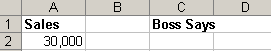Excel - IF Function's 3 Parts

The IF function has three parts:

1. The comparison you will be doing. We are using A2 < 40000
2. What to do if the If statement is true
3. What to do if the If statement is false

If all that information is confusing to you, don't worry, Excel has a built in wizard to help you make your own If functions.

1. Go to the Insert menu and choose Function
2. Select the Logical functions from the drop down selector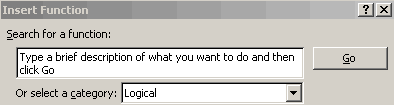3. Choose the IF function and click OK

4.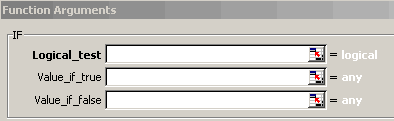5. Put "A2<40000" as the logical expression
6. Put "We are losing money?" as the true value
7. Put "We are making money!" as the false value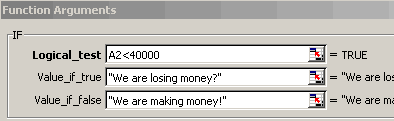8. Press OK

Because our sales were only \$30,000 the if statement should be printing out "We are losing money?"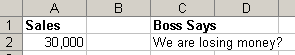This isn't good at all, we're going to get fired!

Let's make some small accounting changes and up that sales figure to \$50,000 and see what the IF function prints out.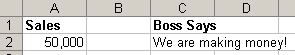Ah that is much better, now we're making money!

Notice that when the value of A2 was \$30,000 it was less than \$40,000 and our IF function was set to print out "We are losing money?" when A2 was less than 40,0000. However, when we changed the value of A2 to \$50,000 the IF statement was now false and it printed out "We are making money!"

Found Something Wrong in this Lesson?

Report a Bug or Comment on This Lesson - Your input is what keeps Tizag improving with time!

 Web Reference HTML Reference CSS Reference CSS Examples PHP Examples Help Tizag Grow Link to Tizag Tizag.com Store
 Advertise Here More Tutorials! Microsoft Office Tutorials Artist Tutorials